// Databricks notebook source exported at Sat, 18 Jun 2016 04:18:07 UTC

# Scalable Data Science

### prepared by Raazesh Sainudiin and Sivanand Sivaram

The html source url of this databricks notebook and its recorded Uji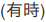for course mechanics, logistics, expectations and course-project suggestions:

NOTE: The links to other notebook may not work in a different shard depending on where you uploaded the ‘scalable-data-science’ archive to! This can be easily fixed by correcting the directory containing ‘scalable-data-science’ folder. Here it is assumed to be in ‘Workspace’ folder.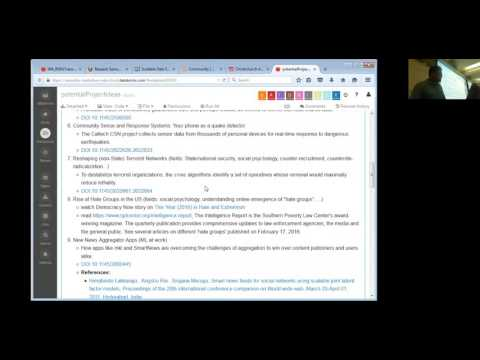and its remaining recorded Uji# Introduction to Spark

## Spark Essentials: RDDs, Transformations and Actions

• This introductory notebook describes how to get started running Spark (Scala) code in Notebooks.
• Working with Spark’s Resilient Distributed Datasets (RDDs)
• creating RDDs
• performing basic transformations on RDDs
• performing basic actions on RDDs

# Spark Cluster Overview:

## Driver Program, Cluster Manager and Worker Nodes

The driver does the following:

1. connects to a cluster manager to allocate resources across applications
• acquire executors on cluster nodes
• executor processs run compute tasks and cache data in memory or disk on a worker node
• sends application (user program built on Spark) to the executors
• sends tasks for the executors to run
• task is a unit of work that will sent to one executor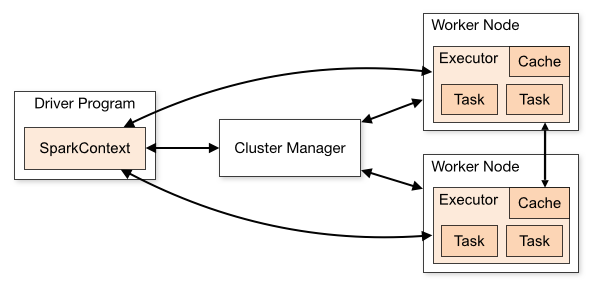See http://spark.apache.org/docs/latest/cluster-overview.html for an overview of the spark cluster. This is embeded in-place below for convenience. Scroll to the bottom to see a Glossary of terms used above and their meanings. You can right-click inside the embedded html `<frame>...</frame>` and use the left and right arrows to navigate within it!

``````
//This allows easy embedding of publicly available information into any other notebook
//when viewing in git-book just ignore this block - you may have to manually chase the URL in frameIt("URL").
//Example usage:
// displayHTML(frameIt("https://en.wikipedia.org/wiki/Latent_Dirichlet_allocation#Topics_in_LDA",250))
def frameIt( u:String, h:Int ) : String = {
"""<iframe
src=""""+ u+""""
width="95%" height="""" + h + """"
sandbox>
<p>
<a href="http://spark.apache.org/docs/latest/index.html">
Fallback link for browsers that, unlikely, don't support frames
</a>
</p>
</iframe>"""
}
displayHTML(frameIt("http://spark.apache.org/docs/latest/cluster-overview.html",700))

``````

## The Abstraction of Resilient Distributed Dataset (RDD)

#### Two types of Operations are possible on an RDD

• Transformations
• Actions

(watch now 2:26):## Transformations

(watch now 1:18):## Actions

(watch now 0:48):Key Points

• Resilient distributed datasets (RDDs) are the primary abstraction in Spark.
• RDDs are immutable once created:
• can transform it.
• can perform actions on it.
• but cannot change an RDD once you construct it.
• Spark tracks each RDD’s lineage information or recipe to enable its efficient recomputation if a machine fails.
• RDDs enable operations on collections of elements in parallel.
• We can construct RDDs by:
• parallelizing Scala collections such as lists or arrays
• by transforming an existing RDD,
• from files in distributed file systems such as (HDFS, S3, etc.).
• We can specify the number of partitions for an RDD
• The more partitions in an RDD, the more opportunities for parallelism
• There are two types of operations you can perform on an RDD:
• transformations (are lazily evaluated)
• map
• flatMap
• filter
• distinct
• actions (actual evaluation happens)
• count
• reduce
• take
• collect
• takeOrdered
• Spark transformations enable us to create new RDDs from an existing RDD.
• RDD transformations are lazy evaluations (results are not computed right away)
• Spark remembers the set of transformations that are applied to a base data set (this is the lineage graph of RDD)
• The allows Spark to automatically recover RDDs from failures and slow workers.
• The lineage graph is a recipe for creating a result and it can be optimized before execution.
• A transformed RDD is executed only when an action runs on it.
• You can also persist, or cache, RDDs in memory or on disk (this speeds up iterative ML algorithms that transforms the initial RDD iteratively).
• Here is a great reference URL for working with Spark.
``````
displayHTML(frameIt("http://spark.apache.org/docs/latest/programming-guide.html",800))

``````

## Let us get our hands dirty in Spark implementing these ideas!

#### Let us look at the legend and overview of the visual RDD Api in the following notebook:

NOTE: The links to other notebook may not work in a different shard depending on where you uploaded the ‘scalable-data-science’ archive to! This can be easily fixed by correcting the directory containing ‘scalable-data-science’ folder. Here it is assumed to be in ‘Workspace’ folder. This NOTE won’t keep reappearing :)### Running Spark

The variable sc allows you to access a Spark Context to run your Spark programs. Recall `SparkContext` is in the Driver Program.*NOTE: Do not create the *sc variable - it is already initialized for you. **

### We will do the following next:

1. Create an RDD using `sc.parallelize`
• Perform the `collect` action on the RDD and find the number of partitions it is made of using `getNumPartitions` action
• Perform the `take` action on the RDD
• Transform the RDD by `map` to make another RDD
• Transform the RDD by `filter` to make another RDD
• Perform the `reduce` action on the RDD
• Transform the RDD by `flatMap` to make another RDD
• Create a Pair RDD
• Perform some transformations on a Pair RDD
• Where in the cluster is your computation running?
• Shipping Closures, Broadcast Variables and Accumulator Variables
• Spark Essentials: Summary
• HOMEWORK

### 1. Create an RDD using `sc.parallelize`

First, let us create an RDD of three elements (of integer type `Int`) from a Scala `Seq` (or `List` or `Array`) with two partitions by using the `parallelize` method of the available Spark Context `sc` as follows:

``````
val x = sc.parallelize(Array(1, 2, 3), 2)    // <Ctrl+Enter> to evaluate this cell (using 2 partitions)

``````
``````
x.  // place the cursor after 'x.' and hit Tab to see the methods available for the RDD x we created

``````

### 2. Perform the `collect` action on the RDD and find the number of partitions it is made of using `getNumPartitions` action

No action has been taken by `sc.parallelize` above. To see what is “cooked” by the recipe for RDD `x` we need to take an action.

The simplest is the `collect` action which returns all of the elements of the RDD as an `Array` to the driver program and displays it.

So you have to make sure that all of that data will fit in the driver program if you call `collect` action!

#### Let us look at the collect action in detail and return here to try out the example codes.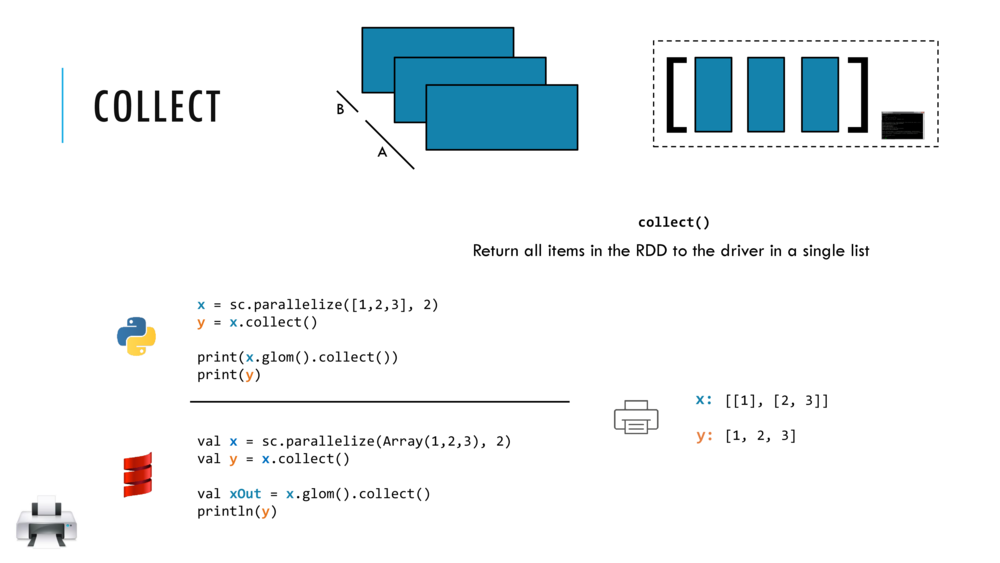Let us perform a `collect` action on RDD `x` as follows:

``````
x.collect()    // <Ctrl+Enter> to collect (action) elements of rdd; should be (1, 2, 3)

``````

CAUTION: `collect` can crash the driver when called upon an RDD with massively many elements.
So, it is better to use other diplaying actions like `take` or `takeOrdered` as follows:

#### Let us look at the getNumPartitions action in detail and return here to try out the example codes.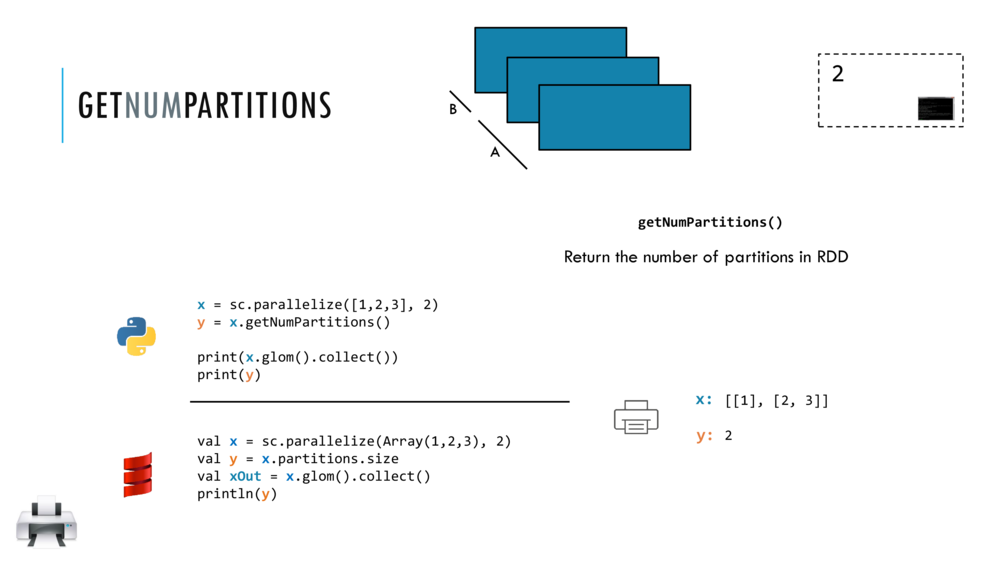``````
// <Ctrl+Enter> to evaluate this cell and find the number of partitions in RDD x
x.getNumPartitions

``````

We can see which elements of the RDD are in which parition by calling `glom()` before `collect()`.

`glom()` flattens elements of the same partition into an `Array`.

``````
x.glom().collect() // glom() flattens elements on the same partition

``````

Thus from the output above, `Array[Array[Int]] = Array(Array(1), Array(2, 3))`, we know that `1` is in one partition while `2` and `3` are in another partition.

##### You Try!

Crate an RDD `x` with three elements, 1,2,3, and this time do not specifiy the number of partitions. Then the default number of partitions will be used. Find out what this is for the cluster you are attached to.

The default number of partitions for an RDD depends on the cluster this notebook is attached to among others - see programming-guide.

``````
val x = sc.parallelize(Seq(1, 2, 3))    // <Shift+Enter> to evaluate this cell (using default number of partitions)

``````
``````
x.getNumPartitions // <Shift+Enter> to evaluate this cell

``````
``````
x.glom().collect() // <Ctrl+Enter> to evaluate this cell

``````

### 3. Perform the `take` action on the RDD

The `.take(n)` action returns an array with the first `n` elements of the RDD.

``````
x.take(2) // Ctrl+Enter to take two elements from the RDD x

``````
##### You Try!

Fill in the parenthes `( )` below in order to `take` just one element from RDD `x`.

``````
x.take(  ) // fill in the parenthesis to take just one element from RDD x and Cntrl+Enter

``````

### 4. Transform the RDD by `map` to make another RDD

The `map` transformation returns a new RDD that’s formed by passing each element of the source RDD through a function (closure). The closure is automatically passed on to the workers for evaluation (when an action is called later).

#### Let us look at the map transformation in detail and return here to try out the example codes.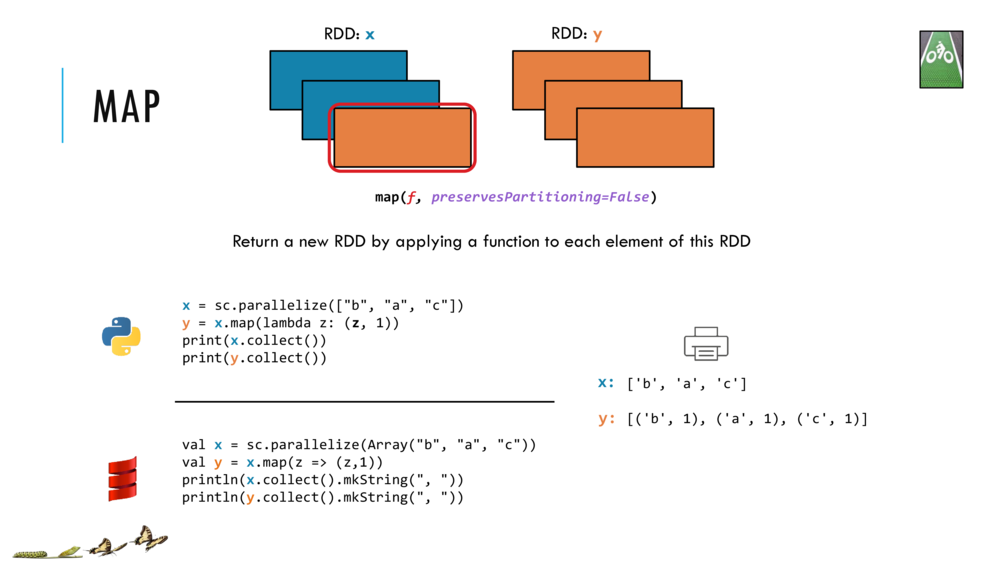``````
// Shift+Enter to make RDD x and RDD y that is mapped from x
val x = sc.parallelize(Array("b", "a", "c")) // make RDD x: [b, a, c]
val y = x.map(z => (z,1))                    // map x into RDD y: [(b, 1), (a, 1), (c, 1)]

``````
``````
// Cntrl+Enter to collect and print the two RDDs
println(x.collect().mkString(", "))
println(y.collect().mkString(", "))

``````

### 5. Transform the RDD by `filter` to make another RDD

The `filter` transformation returns a new RDD that’s formed by selecting those elements of the source RDD on which the function returns `true`.

#### Let us look at the filter transformation in detail and return here to try out the example codes.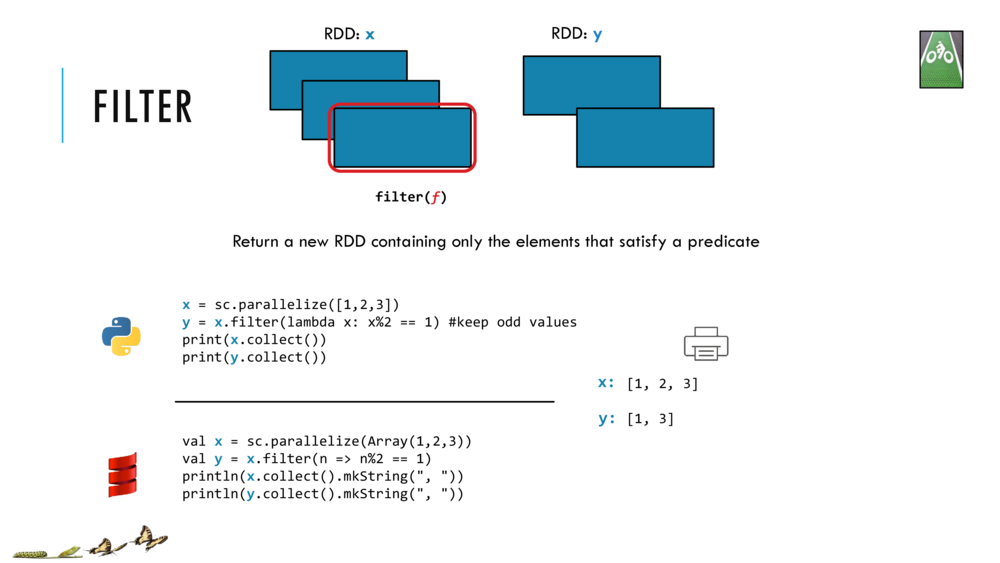``````
//Shift+Enter to make RDD x and filter it by (n => n%2 == 1) to make RDD y
val x = sc.parallelize(Array(1,2,3))
// the closure (n => n%2 == 1) in the filter will
// return True if element n in RDD x has remainder 1 when divided by 2 (i.e., if n is odd)
val y = x.filter(n => n%2 == 1)

``````
``````
// Cntrl+Enter to collect and print the two RDDs
println(x.collect().mkString(", "))
println(y.collect().mkString(", "))

``````

### 6. Perform the `reduce` action on the RDD

Reduce aggregates a data set element using a function (closure). This function takes two arguments and returns one and can often be seen as a binary operator. This operator has to be commutative and associative so that it can be computed correctly in parallel (where we have little control over the order of the operations!).

### Let us look at the reduce action in detail and return here to try out the example codes.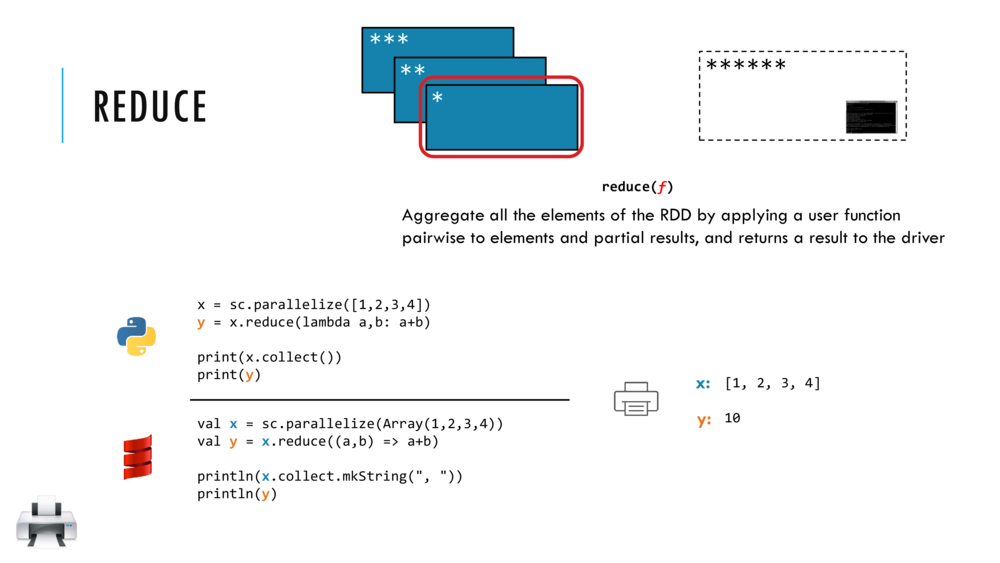``````
//Shift+Enter to make RDD x of inteegrs 1,2,3,4 and reduce it to sum
val x = sc.parallelize(Array(1,2,3,4))
val y = x.reduce((a,b) => a+b)

``````
``````
//Cntrl+Enter to collect and print RDD x and the Int y, sum of x
println(x.collect.mkString(", "))
println(y)

``````

### 7. Transform an RDD by `flatMap` to make another RDD

`flatMap` is similar to `map` but each element from input RDD can be mapped to zero or more output elements. Therefore your function should return a sequential collection such as an `Array` rather than a single element as shown below.

### Let us look at the flatMap transformation in detail and return here to try out the example codes.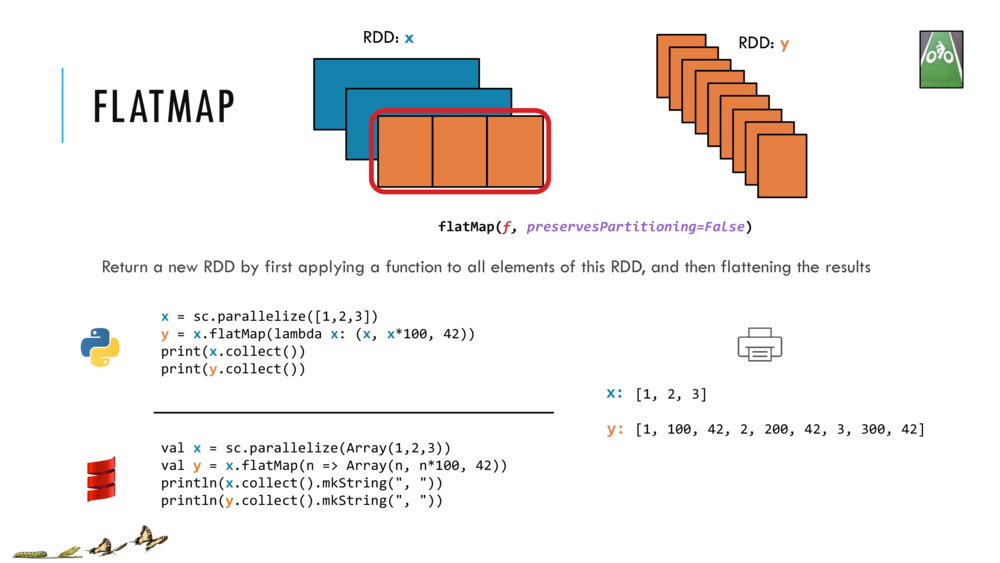``````
//Shift+Enter to make RDD x and flatMap it into RDD by closure (n => Array(n, n*100, 42))
val x = sc.parallelize(Array(1,2,3))
val y = x.flatMap(n => Array(n, n*100, 42))

``````
``````
//Cntrl+Enter to collect and print RDDs x and y
println(x.collect().mkString(", "))
println(y.collect().mkString(", "))

``````

### 8. Create a Pair RDD

Let’s next work with RDD of `(key,value)` pairs called a Pair RDD or Key-Value RDD.

``````
// Cntrl+Enter to make RDD words and display it by collect
val words = sc.parallelize(Array("a", "b", "a", "a", "b", "b", "a", "a", "a", "b", "b"))
words.collect()

``````

Let’s make a Pair RDD called `wordCountPairRDD` that is made of (key,value) pairs with key=word and value=1 in order to encode each occurrence of each word in the RDD `words`, as follows:

``````
// Cntrl+Enter to make and collect Pair RDD wordCountPairRDD
val wordCountPairRDD = words.map(s => (s, 1))
wordCountPairRDD.collect()

``````

### 9. Perform some transformations on a Pair RDD

Let’s next work with RDD of `(key,value)` pairs called a Pair RDD or Key-Value RDD.

Now some of the Key-Value transformations that we could perform include the following.

• `reduceByKey` transformation
• which takes an RDD and returns a new RDD of key-value pairs, such that:
• the values for each key are aggregated using the given reduced function
• and the reduce function has to be of the type that takes two values and returns one value.
• `sortByKey` transformation
• this returns a new RDD of key-value pairs that’s sorted by keys in ascending order
• `groupByKey` transformation
• this returns a new RDD consisting of key and iterable-valued pairs.

Let’s see some concrete examples next.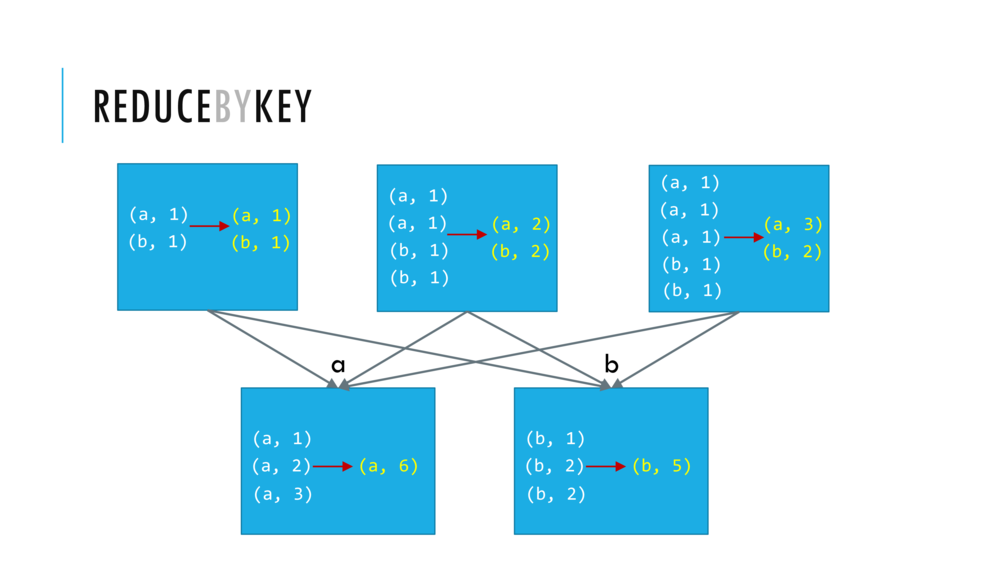``````
// Cntrl+Enter to reduceByKey and collect wordcounts RDD
//val wordcounts = wordCountPairRDD.reduceByKey( _ + _ )
val wordcounts = wordCountPairRDD.reduceByKey( (v1,v2) => v1+v2 )
wordcounts.collect()

``````

Now, let us do just the crucial steps and avoid collecting intermediate RDDs (something we should avoid for large datasets anyways, as they may not fit in the driver program).

``````
//Cntrl+Enter to make words RDD and do the word count in two lines
val words = sc.parallelize(Array("a", "b", "a", "a", "b", "b", "a", "a", "a", "b", "b"))
val wordcounts = words.map(s => (s, 1)).reduceByKey(_ + _).collect()

``````
##### You Try!

You try evaluating `sortByKey()` which will make a new RDD that consists of the elements of the original pair RDD that are sorted by Keys.

``````
// Shift+Enter and comprehend code
val words = sc.parallelize(Array("a", "b", "a", "a", "b", "b", "a", "a", "a", "b", "b"))
val wordCountPairRDD = words.map(s => (s, 1))
val wordCountPairRDDSortedByKey = wordCountPairRDD.sortByKey()

``````
``````
wordCountPairRDD.collect() // Shift+Enter and comprehend code

``````
``````
wordCountPairRDDSortedByKey.collect() // Cntrl+Enter and comprehend code

``````

The next key value transformation we will see is `groupByKey`

When we apply the `groupByKey` transformation to `wordCountPairRDD` we end up with a new RDD that contains two elements. The first element is the tuple `b` and an iterable `CompactBuffer(1,1,1,1,1)` obtained by grouping the value `1` for each of the five key value pairs `(b,1)`. Similarly the second element is the key `a` and an iterable `CompactBuffer(1,1,1,1,1,1)` obtained by grouping the value `1` for each of the six key value pairs `(a,1)`.

CAUTION: `groupByKey` can cause a large amount of data movement across the network. It also can create very large iterables at a worker. Imagine you have an RDD where you have 1 billion pairs that have the key `a`. All of the values will have to fit in a single worker if you use group by key. So instead of a group by key, consider using reduced by key.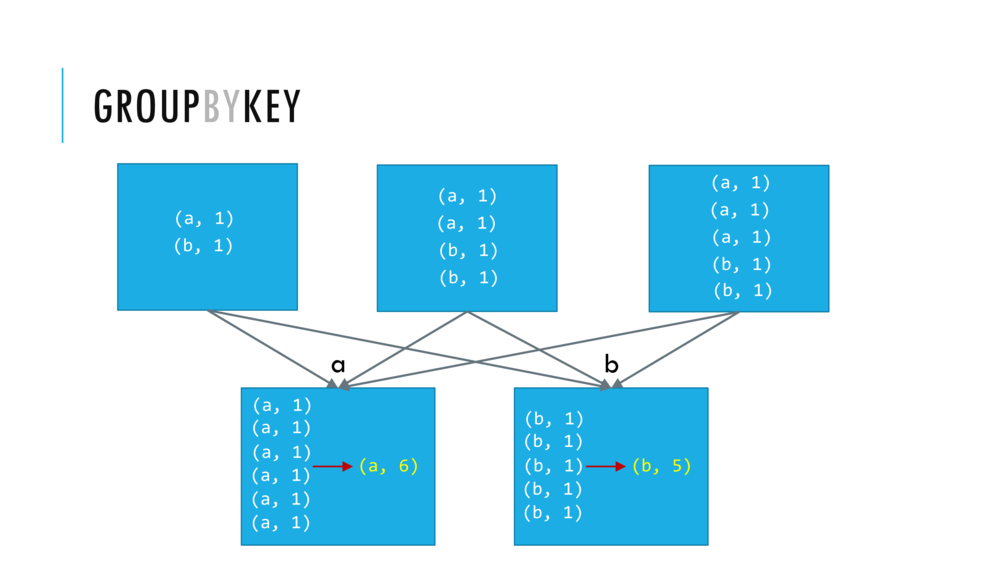``````
val wordCountPairRDDGroupByKey = wordCountPairRDD.groupByKey() // <Shift+Enter> CAUTION: this transformation can be very wide!

``````
``````
wordCountPairRDDGroupByKey.collect()  // Cntrl+Enter

``````

### 10. Where in the cluster is your computation running?

``````
val list = 1 to 10
var sum = 0
list.foreach(x => sum = sum + x)
print(sum)

``````
``````
val rdd = sc.parallelize(1 to 10)
var sum = 0
rdd.foreach(x => sum = sum + x)
rdd.collect
print(sum)

``````

### 11. Shipping Closures, Broadcast Variables and Accumulator Variables

#### Closures, Broadcast and Accumulator Variables

(watch now 2:06):We will use these variables in the sequel.

#### SUMMARY

Spark automatically creates closures

• for functions that run on RDDs at workers,
• and for any global variables that are used by those workers
• one closure per worker is sent with every task
• and there’s no communication between workers
• closures are one way from the driver to the worker
• any changes that you make to the global variables at the workers
• are not sent to the driver or
• are not sent to other workers.

The problem we have is that these closures

• are automatically created are sent or re-sent with every job
• with a large global variable it gets inefficient to send/resend lots of data to each worker
• we cannot communicate that back to the driver

To do this, Spark provides shared variables in two different types.

• lets us to efficiently send large read-only values to all of the workers
• these are saved at the workers for use in one or more Spark operations.
• accumulator variables
• These allow us to aggregate values from workers back to the driver.
• only the driver can access the value of the accumulator
• for the tasks, the accumulators are basically write-only

### 12. Spark Essentials: Summary (watch now: 0:29)NOTE: In databricks cluster, we (the course coordinator/administrators) set the number of workers for you.

### 13. HOMEWORK

See the notebook in this folder named `005_RDDsTransformationsActionsHOMEWORK`. This notebook will give you more examples of the operations above as well as others we will be using later, including:

• Perform the `takeOrdered` action on the RDD
• Transform the RDD by `distinct` to make another RDD and
• Doing a bunch of transformations to our RDD and performing an action in a single cell.

### Importing Standard Scala and Java libraries

• For other libraries that are not available by default, you can upload other libraries to the Workspace.
• Refer to the Libraries guide for more details.
``````
import scala.math._
val x = min(1, 10)

``````
``````
import java.util.HashMap
val map = new HashMap[String, Int]()
map.put("a", 1)
map.put("b", 2)
map.put("c", 3)
map.put("d", 4)
map.put("e", 5)

``````

Updated: